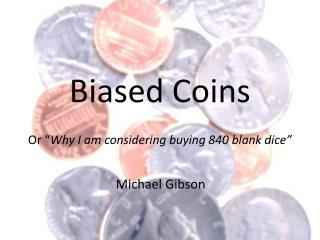DownloadDownload PresentationBiased Coins

# Biased Coins

Télécharger la présentation## Biased Coins

- - - - - - - - - - - - - - - - - - - - - - - - - - - E N D - - - - - - - - - - - - - - - - - - - - - - - - - - -
##### Presentation Transcript

1. Biased Coins Or “Why I am considering buying 840 blank dice” Michael Gibson

2. Some “QI” Questions… What is the total value of the coins in this bag? (I am holding up a bag which appears to contain 6 10p coins.) If a coin is tossed once and shows heads, what is the probability that it will show heads next time? What if the coin was chosen from a collection with a uniform distribution of biases?

3. Defining Bias… We can define the bias of a coin by its weighting, , i.e. the probability of heads on any toss. We consider an infinite collection of coins with a uniform distribution of weightings, i.e. if , otherwise. This will later be referred to as “our model”.

4. Outcomes for 1 or 2 tosses

5. Results for 1 or 2 tosses Interestingly, we find To answer our original question, we can use Bayes’ Theorem:

6. Extension to r heads from n tosses We will use the shorthand “r/n” for this. Binomial theory gives: For a randomly selected coin, we have What if we toss a coin n times, resulting in r heads? What is the probability that we get heads on the next toss? Using Bayes’ Theorem again, it can be shown that Spend a moment thinking about the meaning of this result…

7. Replication With 2 Coins? Suppose we have just 2 coins in the collection, but can choose their biases to be We require which gives . It can be shown that this actually replicates our model perfectly for up to three tosses of the chosen coin. For example, Of course, producing coins with this exact weighting would be very difficult!

8. Physical Replication With Actual Coins Suppose we have coins, fair, double-headed and double-tailed. We require Which leads to . So will work! if you have a collection of coins from which you repeatedly choose a coin at random, toss it up to 3 times, then return it to the collection, the results obtained from a collection consisting of 4 fair coins, 1 double-headed coin and 1 double-tailed coin are statistically indistinguishable from those that would be obtained from an infinite collection of coins with a continuous uniform distribution of biases.

9. Let’s Keep Going! OK so we’ve done all we realistically can with coins, but we can go further if we simulate coins using 6-sided dice with “H” or “T” written on their faces. There are 7 different biases possible now. We will restrict ourselves to collections with a symmetric distribution, described by the vector as follows:

10. Let’s Keep Going! If we initially restrict ourselves to only 2 non-zero components, then we find the following vectors (and their multiples) work for up to 3throws: (2, 0, 3, 0) (4, 0, 0, 1) * note this is equivalent to our coins earlier (0, 1, 2, 0) (0, 3, 0, 1) Any (physically possible) linear combination of these will also work for at least 3 throws (but note that these 4 vectors can’t all be linearly independent, for the same reason). It turns out that adding the first 3 together to give (6, 1, 5, 1) actually works for 5 throws, as does the vector (52, 27, 54, 13), or any linear combination these two. The former seems optimal.

11. Let’s Keep Going! If you have a collection of six sided dice, representing coins by having either heads or tails marked on each face, in the ratio H:T, from which you repeatedly choose one at random, roll it up to 5 times, then return it to the collection, the results obtained from a collection consisting of 20 dice as detailed below are statistically indistinguishable from those that would be obtained from an infinite collection of coins with a continuous uniform distribution of biases.

12. Let’s Keep Going! We still have two linearly independent vectors to combine, so this should give us hope of going further still. There is only one vector (plus its multiples) which will work, and it turns out to be (272, 27, 216, 41). This matches our model for up to 7throws.

13. Let’s Keep Going! If you have a collection of six sided dice, representing coins by having either heads or tails marked on each face, in the ratio H:T, from which you repeatedly choose one at random, roll it up to 7 times, then return it to the collection, the results obtained from a collection consisting of 840 dice as detailed below are statistically indistinguishable from those that would be obtained from an infinite collection of coins with a continuous uniform distribution of biases.

14. And that is why I am considering buying 840 blank dice. Anyone fancy chipping in?